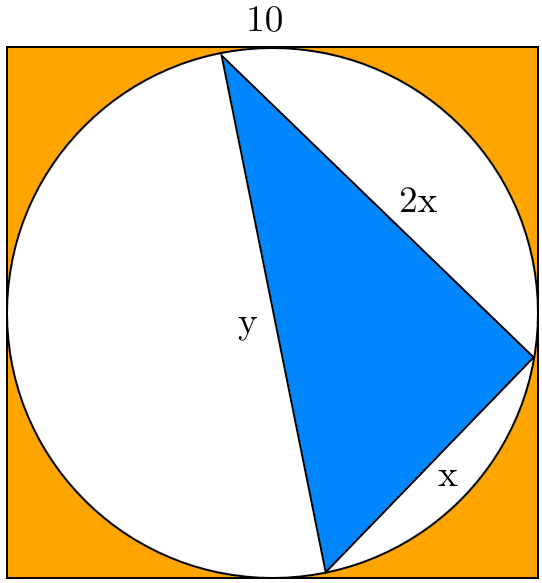# Difference

Geometry Level 3The diagram above shows a circle inscribed inside a square of side length $10$, which has a right triangle of legs length $x$ and $2x$, and hypotenuse length $y$ inscribed in it.

The difference between the area of the $\color{#ee7600}{\text{orange}}$ shaded region and the $\color{#3D99F6}{\text{blue}}$ shaded region can be written as $a(b-a\pi )$ for integers $a$ and $b$. Let the digit sum of $b$ be $B$. Then find the remainder of $\large\overline { Baa } \div B$

×

Problem Loading...

Note Loading...

Set Loading...